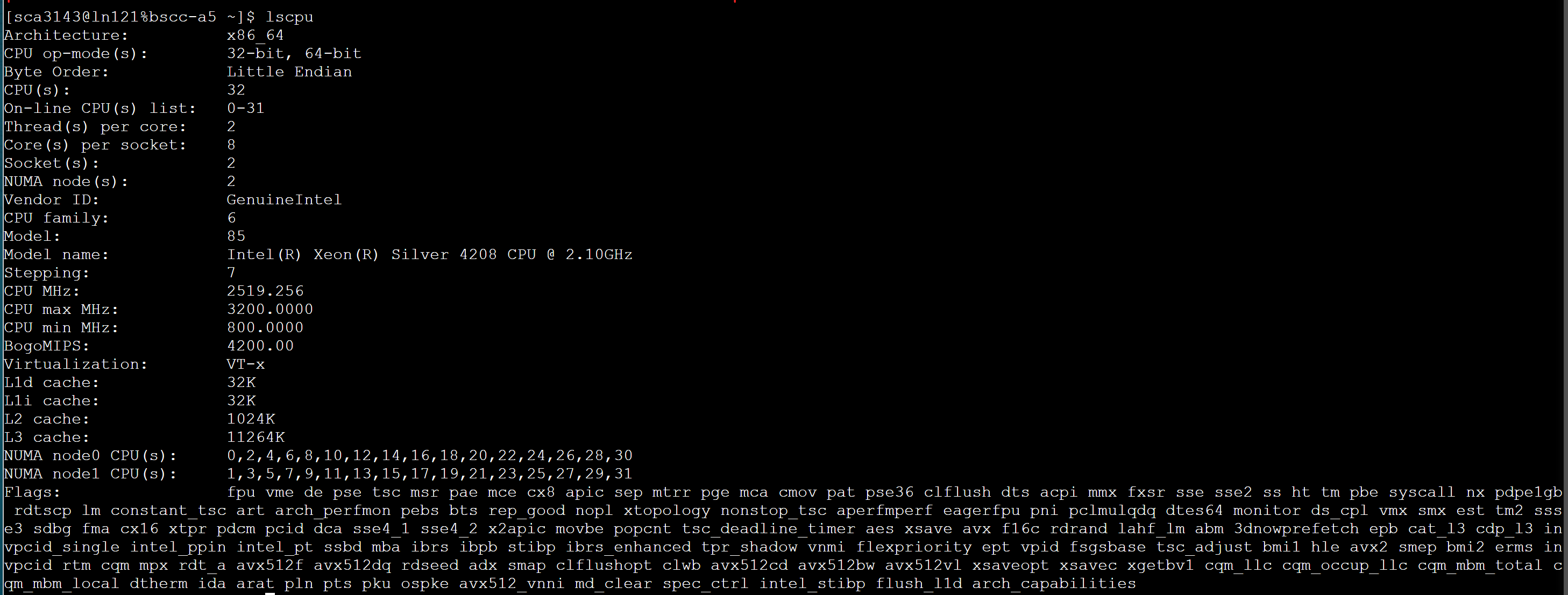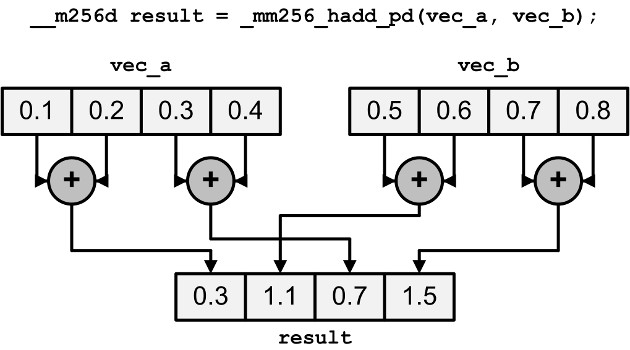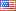## 1.文章来源

Matt Scarpino（USA）

Crunching Numbers with AVX and AVX2 - CodeProject

## 2.介绍

2008年，英特尔推出了一套新的高性能指令，称为高级向量扩展(AVX)。AVX执行许多与SSE指令相同的操作，但以更快的速度对更大的数据块进行操作。最近，英特尔在AVX2和AVX512系列中发布了额外的指令。本文的重点是通过称为intrinsic funtions的特殊C函数访问AVX和AVX2指令。

1. Initialization intrinscis
2. Arithmetic intrinsics
3. Permute/shuffle intrinsics

## 3.基本要求

• Intel’s Sandy Bridge/Sandy Bridge E/Ivy Bridge/Ivy Bridge E
• AMD’s Bulldozer/Piledriver/Steamroller/Excavator

• AMD’s Excavator

[个人补充]## 4.向量化概述

AVX指令通过同时处理大块值而不是单独处理值来提高应用程序的性能。这些值大块称为向量，AVX向量最多可以包含256位数据。

## 5.AVX编程基础

• Data types
• Function naming conventions
• Compiling AVX applications

### 5.1数据类型

Table 1:AVX/AVX2 Data Types

Data TypeDescription
`__m128`128-bit vector containing 4 `float`s
`__m128d`128-bit vector containing 2 `double`s
`__m128i`128-bit vector containing integers
`__m256`256-bit vector containing 8 `float`s
`__m256d`256-bit vector containing 4 `double`s
`__m256i`256-bit vector containing integers

### 5.3函数命名约定

AVX/AVX2 intrinsics的名称一开始可能令人困惑，但命名约定确是非常直白的。一旦你理解了它，你就可以通过看它的名字来大致判断一个函数是做什么的。AVX/AVX2 intrinsics的一般形式如下:

_mm__

1. `<bit_width>` identifies the size of the vector returned by the function. For 128-bit vectors, this is empty. For 256-bit vectors, this is set to `256`.
2. `<name>` describes the operation performed by the intrinsic
3. `<data_type>` identifies the data type of the function’s primary arguments

• `ps` - vectors contain `float`s (`ps` stands for packed single-precision)
• `pd` - vectors contain `double`s (`pd` stands for packed double-precision)
• `epi8/epi16/epi32/epi64` - vectors contain 8-bit/16-bit/32-bit/64-bit signed integers
• `epu8/epu16/epu32/epu64` - vectors contain 8-bit/16-bit/32-bit/64-bit unsigned integers
• `si128`/`si256` - unspecified 128-bit vector or 256-bit vector
• `m128/m128i/m128d/m256/m256i/m256d` - identifies input vector types when they’re different than the type of the returned vector

AVX数据类型以两个下划线和一个m开头。函数以一个下划线和两个m开头。我很容易搞混这一点，所以我想出了一种方法来记住它们的区别:数据类型代表内存（memory），函数代表多媒体操作（multimedia）。这是我能做的最好的了。

### 5.4构建AVX应用程序

hello_avx.c中的代码显示了一个基本的AVX应用程序的样子:

[这就是一个简单的向量减法例子，大家可以对应数据看一下]

### 5.5初始化intrinsics

#### 5.5.1使用标量值初始化

AVX提供了将一个或多个值组合成256位向量的intrinsics funtions。表2列出了它们的名称，并提供了每个名称的描述。也有类似的intrinsics初始化128位向量，但它们是由SSE提供的，而不是AVX。函数名的唯一区别是_mm256_被替换为_mm_。

Table 2: Initialization Intrinsics

FunctionDescription
`_mm256_setzero_ps/pd`Returns a floating-point vector filled with zeros
`_mm256_setzero_si256`Returns an integer vector whose bytes are set to zero
`_mm256_set1_ps/pd`Fill a vector with a floating-point value
`_mm256_set1_epi8/epi16` `_mm256_set1_epi32/epi64`Fill a vector with an integer
`_mm256_set_ps/pd`Initialize a vector with eight floats (ps) or four doubles (pd)
`_mm256_set_epi8/epi16` `_mm256_set_epi32/epi64`Initialize a vector with integers
`_mm256_set_m128/m128d/` `_mm256_set_m128i`Initialize a 256-bit vector with two 128-bit vectors
`_mm256_setr_ps/pd`Initialize a vector with eight floats (ps) or four doubles (pd) in reverse order
`_mm256_setr_epi8/epi16` `_mm256_setr_epi32/epi64`Initialize a vector with integers in reverse order

#### 5.5.2从内存加载数据

AVX/AVX2的一个常见用法是将数据从内存加载到向量中，对向量进行处理，并将结果存储回内存。第一步是使用表3中列出的intrinsic funtions完成的。最后两个函数前面有(2)，因为它们是由AVX2而不是AVX提供的。

Data TypeDescription
`_mm256_load_ps/pd`Loads a floating-point vector from an aligned memory address
`_mm256_load_si256`Loads an integer vector from an aligned memory address
`_mm256_loadu_ps/pd`Loads a floating-point vector from an unaligned memory address
`_mm256_loadu_si256`Loads an integer vector from an unaligned memory address
`_mm_maskload_ps/pd` `_mm256_maskload_ps/pd`Load portions of a 128-bit/256-bit floating-point vector according to a mask
`(2)_mm_maskload_epi32/64` `(2)_mm256_maskload_epi32/64`Load portions of a 128-bit/256-bit integer vector according to a mask

【个人补充】关于内存对齐以及相关函数

AVX向量化学习(二)-内存对齐的应用 - Amicoyuan (xingyuanjie.top)

【int型在计算机的存储是补码，正数的补码最高位为0，所以这里返回0，负数的补码最高位为1，所以这里返回的是内存中相应的元素】

1. 代码使用_setr_函数而不是_set_来设置掩码向量的内容，因为它在将向量元素传递给函数时对它们进行排序。
2. 负整数的最高位总是1。这就是掩码向量包含五个负数和三个正数的原因。

## 6.Arithmetic Intrinsics

### 6.1加法和减法

Table 4: Addition and Subtraction Intrinsics

Data TypeDescription
`_mm256_add_ps/pd`Add two floating-point vectors
`_mm256_sub_ps/pd`Subtract two floating-point vectors
`(2)_mm256_add_epi8/16/32/64`Add two integer vectors
`(2)_mm236_sub_epi8/16/32/64`Subtract two integer vectors
`(2)_mm256_adds_epi8/16` `(2)_mm256_adds_epu8/16`Add two integer vectors with saturation
`(2)_mm256_subs_epi8/16` `(2)_mm256_subs_epu8/16`Subtract two integer vectors with saturation
`_mm256_hadd_ps/pd`Add two floating-point vectors horizontally
`_mm256_hsub_ps/pd`Subtract two floating-point vectors horizontally
`(2)_mm256_hadd_epi16/32`Add two integer vectors horizontally
`(2)_mm256_hsub_epi16/32`Subtract two integer vectors horizontally
`(2)_mm256_hadds_epi16`Add two vectors containing shorts horizontally with saturation
`(2)_mm256_hsubs_epi16`Subtract two vectors containing shorts horizontally with saturation
`_mm256_addsub_ps/pd`Add and subtract two floating-point vectors

• 如果用_mm256_sub_epi16减去这些值，饱和度将被忽略，存储的结果将是28,536 (0x6F78)。
• 如果用_mm256_subs_epi16减去这些值，结果将被压缩到最小值-32,768 (0x8000)。Figure 1: Horizontal Addition of Two Vectors

### 6.2乘法和除法

Table 5: Multiplication and Division Intrinsics

Data TypeDescription
`_mm256_mul_ps/pd`Multiply two floating-point vectors
`(2)_mm256_mul_epi32/` `(2)_mm256_mul_epu32`Multiply the lowest four elements of vectors containing 32-bit integers
`(2)_mm256_mullo_epi16/32`Multiply integers and store low halves
`(2)_mm256_mulhi_epi16/` `(2)_mm256_mulhi_epu16`Multiply integers and store high halves
`(2)_mm256_mulhrs_epi16`Multiply 16-bit elements to form 32-bit elements
`_mm256_div_ps/pd`Divide two floating-point vectors

___m256i _mm256_mul_epi32 (m256i a, __m256i b)

Description

Multiply the low signed 32-bit integers from each packed 64-bit element in a and b, and store the signed 64-bit results in dst.

Operation

_mullo_函数类似于整数_mul_函数，但它们不是乘低元素，而是乘两个向量的每个元素，只存储每个乘积的低一半。

Synopsis

m256i _mm256_mullo_epi32 (m256i a, __m256i b)
#include <immintrin.h>
Instruction: vpmulld ymm, ymm, ymm
CPUID Flags: AVX2

Description

Multiply the packed signed 32-bit integers in a and b, producing intermediate 64-bit integers, and store the low 32 bits of the intermediate integers in dst.

Operation

_mm256_mulhi_epi16和_mm256_mulhi_epu16 intrinsics类似，但是它们存储整数积的高一半。

Synopsis

m256i _mm256_mulhi_epi16 (m256i a, __m256i b)
#include <immintrin.h>
Instruction: vpmulhw ymm, ymm, ymm
CPUID Flags: AVX2

Description

Multiply the packed signed 16-bit integers in a and b, producing intermediate 32-bit integers, and store the high 16 bits of the intermediate integers in dst.

Operation

### 6.3Fused Multiply and Add (FMA)

AVX2提供了将乘法和加法融合在一起的指令。也就是说，它们不是返回整数(整数(a * b) + c)，而是返回整数(a * b + c)。因此，这些指令比分别执行乘法和加法提供了更高的速度和准确性【这里正是FMA的特点】。

Table 6: FMA Intrinsics

Data TypeDescription
`(2)_mm_fmadd_ps/pd/` `(2)_mm256_fmadd_ps/pd`Multiply two vectors and add the product to a third (res = a * b + c)
`(2)_mm_fmsub_ps/pd/` `(2)_mm256_fmsub_ps/pd`Multiply two vectors and subtract a vector from the product (res = a * b - c)
`(2)_mm_fmadd_ss/sd`Multiply and add the lowest element in the vectors (res = a * b + c)
`(2)_mm_fmsub_ss/sd`Multiply and subtract the lowest element in the vectors (res = a * b - c)
`(2)_mm_fnmadd_ps/pd` `(2)_mm256_fnmadd_ps/pd`Multiply two vectors and add the negated product to a third (res = -(a * b) + c)
`(2)_mm_fnmsub_ps/pd/` `(2)_mm256_fnmsub_ps/pd`Multiply two vectors and add the negated product to a third (res = -(a * b) - c)
`(2)_mm_fnmadd_ss/sd`Multiply the two lowest elements and add the negated product to the lowest element of the third vector (res = -(a * b) + c)
`(2)_mm_fnmsub_ss/sd`Multiply the lowest elements and subtract the lowest element of the third vector from the negated product (res = -(a * b) - c)
`(2)_mm_fmaddsub_ps/pd/` `(2)_mm256_fmaddsub_ps/pd`Multiply two vectors and alternately add and subtract from the product (res = a * b - c)
`(2)_mm_fmsubadd_ps/pd/` `(2)_mmf256_fmsubadd_ps/pd`Multiply two vectors and alternately subtract and add from the product (res = a * b - c)

Synopsis

__m256d _mm256_fnmadd_pd (m256d a, m256d b, m256d c)
#include <immintrin.h>
CPUID Flags: FMA

Description

Multiply packed double-precision (64-bit) floating-point elements in a and b, add the negated intermediate result to packed elements in c, and store the results in dst.

Operation

FMA指令是由AVX2提供的，因此您可能认为使用gcc构建应用程序需要使用-mavx2标志。但是我发现-mfma标志是必需的。否则，我会得到奇怪的编译错误。

## 7.Permuting and Shuffling

AVX/AVX2为此目的提供了许多intrinsic funtion，其中两大类是_permute_函数和_shuffle_函数。本节介绍这两种类型的intrinsic。

### 7.1Permuting

AVX提供了返回一个向量的函数，该向量包含一个向量的重新排列的元素。表7列出了这些排列函数，并提供了对每个函数的描述。

Table 7: Permute Intrinsics

Data TypeDescription
`_mm_permute_ps/pd/` `_mm256_permute_ps/pd`Select elements from the input vector based on an 8-bit control value
`(2)_mm256_permute4x64_pd/` `(2)_mm256_permute4x64_epi64`Select 64-bit elements from the input vector based on an 8-bit control value
`_mm256_permute2f128_ps/pd`Select 128-bit chunks from two input vectors based on an 8-bit control value
`_mm256_permute2f128_si256`Select 128-bit chunks from two input vectors based on an 8-bit control value
`_mm_permutevar_ps/pd` `_mm256_permutevar_ps/pd`Select elements from the input vector based on bits in an integer vector
`(2)_mm256_permutevar8x32_ps`/ `(2)_mm256_permutevar8x32_epi32`Select 32-bit elements (`float`s and `int`s) using indices in an integer vector

_permute_ intrinsic接受两个参数:一个输入向量和一个8位控制值。控制值的位决定输入向量的哪个元素插入到输出中。

Figure 4: Operation of the Permute Intrinsic Function

_permutevar_ intrinsic执行与_permute_ intrinsic相同的操作。但是它们不是使用8位控制值来选择元素，而是依赖于与输入向量大小相同的整数向量。例如，_mm256_permute_ps的输入向量是_mm256，因此整数向量是_mm256i。整数向量的高位执行选择的方式与_permute_ intrinsic的8位控制值的位相同。

### 7.2Shuffling

Table 8: Shuffle Intrinsics

Data TypeDescription
`_mm256_shuffle_ps/pd`Select floating-point elements according to an 8-bit value
`_mm256_shuffle_epi8/` `_mm256_shuffle_epi32`Select integer elements according to an 8-bit value
`(2)_mm256_shufflelo_epi16/` `(2)_mm256_shufflehi_epi16`Select 128-bit chunks from two input vectors based on an 8-bit control value

Synopsis

m256 _mm256_shuffle_ps (m256 a, __m256 b, const int imm8)
#include <immintrin.h>
Instruction: vshufps ymm, ymm, ymm, imm8
CPUID Flags: AVX

Description

Shuffle single-precision (32-bit) floating-point elements in a within 128-bit lanes using the control in imm8, and store the results in dst.

Operation

## 8.Complex Multiplication

【图片丢失】

Figure 6: Complex Multiplication Using Vectors

1. 将vec1和vec2相乘，并将结果存储在vec3中。
2. 切换vec2的实/虚值。
3. 求vec2的虚数的负数。
4. 将vec1和vec2相乘，并将结果存储在vec4中。
5. 对vec3和vec4进行水平相减，得到vec1中的答案。

complex_multi .c中的代码展示了如何使用AVX intrinsic执行此操作:

## 10.History

2/20 - Fixed formatting and image links

4/2 - Fixed a couple typographical errorsUnited States